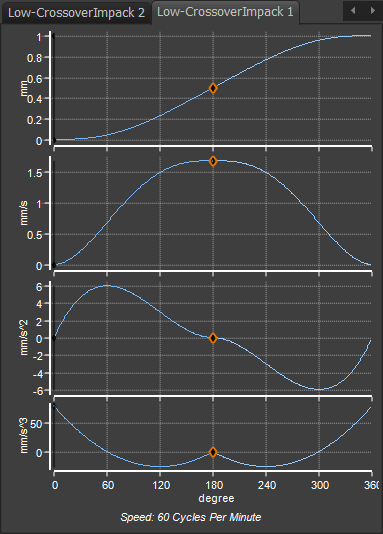﻿ MotionDesigner Reference & User Interface > Motion Laws / Cam-Laws for Segments > Low Impact Crossover Motion-Law

# Low Impact Crossover Motion-Law

### Low Impact at Crossover.

#### Impact, Overrun, and Crossover.

Crossover is the timing at which acceleration becomes a deceleration, and vice versa.

At crossover, machine components traverse backlash to impact with each other.

As the Torque reverses, the load may drive the motor. The drive motor may accelerate so that, for a short period, the motor rotates faster than intended. This is called overrun. As a result, the velocity and acceleration of the cam-follower may also be greater than intended.

A motion-law with Low Jerk at Crossover means the acceleration change less rapidly at crossover - which also means the Jerk is low at crossover. As the acceleration is low for a longer period, the velocity changes less rapidly, to reduce Impacts and Overrun.

#### Low Impact at Crossover.

Low or Zero Impact at Crossover motion-law is not available in the Motion-Law Selector. Use two Flexible Polynomial motion-laws.

Velocity and Acceleration are zero at the start and end.

Acceleration and Jerk are zero at the mid-point.

The Low Impact at Crossover is typically symmetrical. You can make it asymmetrical if you move the X-axis and the Position at the mid-point. The maximum Velocity should not change, but the maximum Acceleration and Jerk values increase.

Motion-Law Coefficients

 Velocity Coefficient : Acceleration Coefficient : Jerk Coefficient : Jerk at Crossover :

#### Two Flexible Polynomial segments to give Low Jerk at Crossover motion-law.Low Jerk at Crossover (with two Flexible-Polynomial Segments)

Motion-Values of each motion-derivative - Normalized values

Start of rise segment 1 :

X = 0 ( 0º )

Position = 0

Velocity = 0

Acceleration = 0

Jerk = Unspecified ( Actual value = 80 mm//s/s/s )

Mid-Point :

X = 0.5 (180º )

Position = 0.5

Velocity = Unspecified ( Actual value = 1.6667 mm/s )

Acceleration = 0

Jerk = 0

End of return Segment 2 :

X = 1 ( 360º )

Position = 1

Velocity = 0

Acceleration = 0

Jerk = Unspecified  ( Actual value = 80 mm//s/s/s )